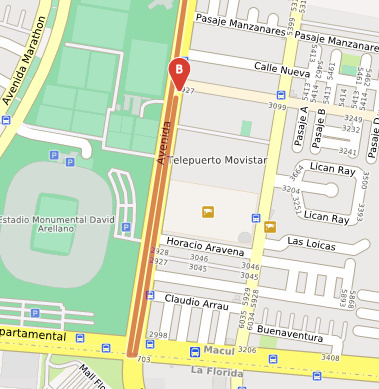# Matrix Distance vs Route distance

Hi, im testing matrix api, and find a little difference between the matrix result and route result.
for example:

from : -70.60336, -33.504066
to : -70.609194, -33.452779

in Matrix the result is : 8.05 km

but when im use the route from same points the distance is : 7.3Km

in both cases im using driving-car option.

I have two questions.

First : Why that difference?

Second : how can i know what route is selected in matrix result?

regards.

Hi,
Second question: You cannot get the route. The matrix calculation does only provide times and distances, no routes.

hi, you cant test with :
Route : (7.26 km)
https://maps.openrouteservice.org/directions?n1=-33.50407&n2=-70.603359&n3=17&a=-33.452756,-70.609089,-33.504066,-70.60336&b=0&c=0&k1=en-US&k2=km

matrix Opt1 : (8.05 km )

``````  curl -X POST \
'https://api.openrouteservice.org/v2/matrix/driving-car' \
-H 'Content-Type: application/json' \
-H 'Accept: application/json, application/geo+json, application/gpx+xml, img/png; charset=utf-8' \
-H 'Authorization: %%APIKEY%%' \
-d '{"locations":[[-70.6033605,-33.5040664],[-70.609089,-33.452756]],"destinations":,"metrics":["distance","duration"],"resolve_locations":"true","units":"km"}'
``````

matrix Opt2: ( 7.26 km )

``````  curl -X POST \
'https://api.openrouteservice.org/v2/matrix/driving-car' \
-H 'Content-Type: application/json' \
-H 'Accept: application/json, application/geo+json, application/gpx+xml, img/png; charset=utf-8' \
-H 'Authorization:  %%APIKEY%%' \
-d '{"locations":[[-70.60336,-33.504066],[-70.609089,-33.452756]],"destinations":,"metrics":["distance","duration"],"units":"km"}'
``````

Ok. i found the reason for that difference.

when i use the route, the latlon change from -33.5040664,-70.6033605 to -33.504066, -70.60336.

then in matrix its the same , if i use the changed latlon i receive 7.26km, but if i use the original latlon ( -33.5040664,-70.6033605), the result is 8.05Km.

if im not wrong, from Lon : -70.6033605 to -70.60336. is almost 1 meter, but in matrix calculation that meter means 1 km.
the change occurs in -70.6033601. from -70.6033600 to -70.6033601 gives me 7.26 but from -70.6033602 to -70.6033605 gives me 8.5

what do you recommend me? use 5, 6 or 7 decimals for matrix??

regardsMoving your second marker just slightly south means that the tour has to go south and make a u-turn instead of going the other way, as you can see on the image.
This is due to the slight difference in coordinates. That is how you get the 1km difference.
Use the number of decimals that is sufficient for you!